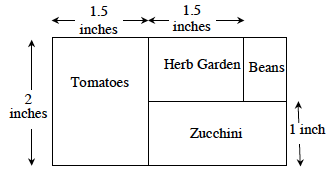### Home > CC2MN > Chapter 4 > Lesson 4.4.3 > Problem4-156

4-156.

Hank is planning his vegetable garden. He has created the scale drawing below. He is planning for the actual area for the tomatoes to be $12$ feet by $9$ feet. All angles are right angles.

1.How many feet in the garden does each inch on the drawing represent?

If the actual area for the tomatoes is $12$ feet by $9$ feet, and the scaled drawing is $2$ inches by $1.5$ inches, you can write a ratio comparing the dimensions of the actual garden to the dimensions of the scaled drawing.

$\frac{\text{12 feet}}{\text{2 inches}} = \frac{x\text{ feet}}{\text{1 inch}}$

Solve for $x$.

$x=6$

2. What are the length and width of the herb garden on the drawing in inches?

Since you already know the length of the herb garden, all you need to do is find the width.

By examining the diagram, you can tell that the width of the herb garden is the width of the zucchini section subtracted from the width of the tomato section.

3. What are the length and width of the real herb garden in feet?

In part (b), you found that the dimensions of the herb garden in the drawing are $1.5$ in by $1$ in.
Using the scale factor found in part (a), what are the dimensions of the real herb garden?

$9$ ft by $6$ ft

4. What is the area of the real herb garden (in square feet)?

The area of a rectangular figure is its length multiplied by its width.

$54$ sq ft

5. Remember that there are $12$ inches in one foot. If Hank measures the real herb garden in square inches instead of square feet, what will its area be?

First convert the dimensions of the real herb garden into inches by using the ratio $12$ in:1ft.

$9$ ft by $6$ ft → $108$ in by $72$ in

How can you use a figure's dimensions to find its area? See part (d).